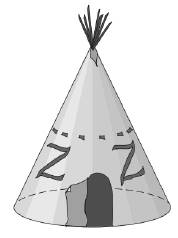Chapter 9.3, Problem 24EElementary Geometry For College St...

7th Edition
Alexander + 2 others
ISBN: 9781337614085

Solutions

Chapter
SectionElementary Geometry For College St...

7th Edition
Alexander + 2 others
ISBN: 9781337614085
Textbook Problem

Rukia discovers that the teepee with a circular floor has a radius length of 6 ft and a height of 15 ft. Find the volume of the enclosure.To determine

To find:

The volume of the enclosure.

Explanation

Given:

Rukia discovers that the teepee has a circular floor with a radius equal to 6 ft and a height of 15 ft.

Formula used:

The volume of the right circular cone is V=13πr2h.

Calculation:

Given, r=6ft and h=15ft.

Substitute the known values in the formula,

Still sussing out bartleby?

Check out a sample textbook solution.

See a sample solution

The Solution to Your Study Problems

Bartleby provides explanations to thousands of textbook problems written by our experts, many with advanced degrees!

Get Started

Find more solutions based on key concepts